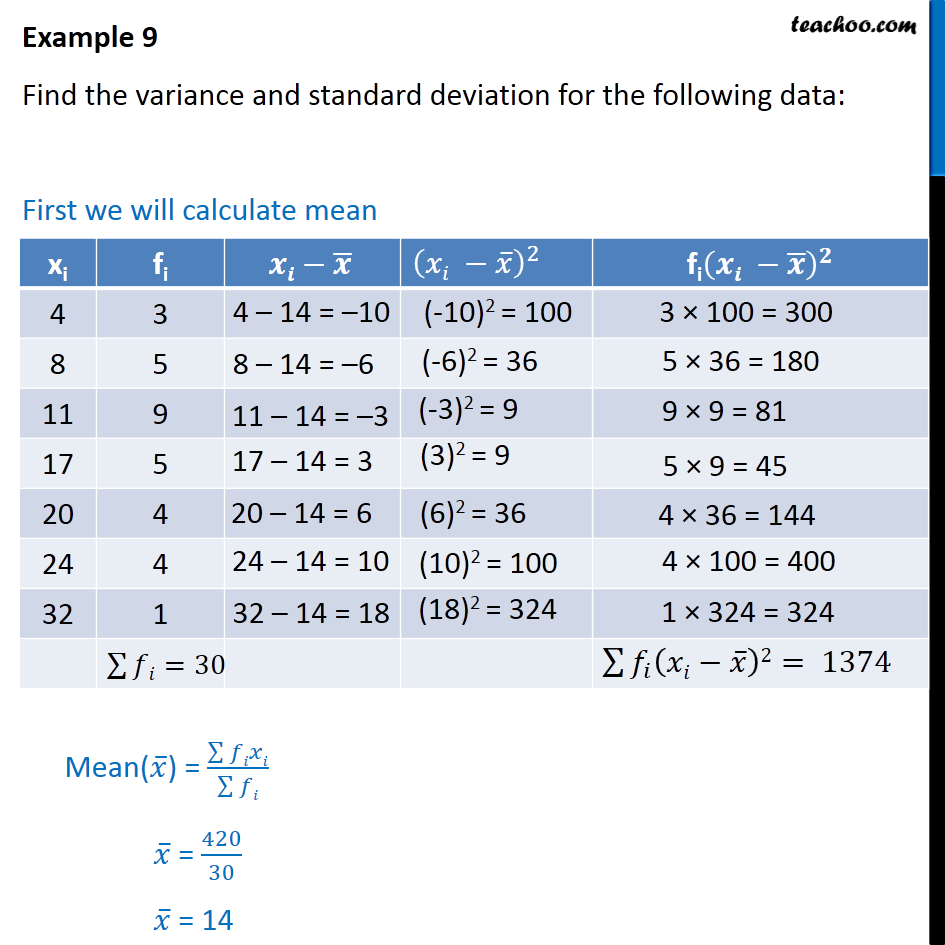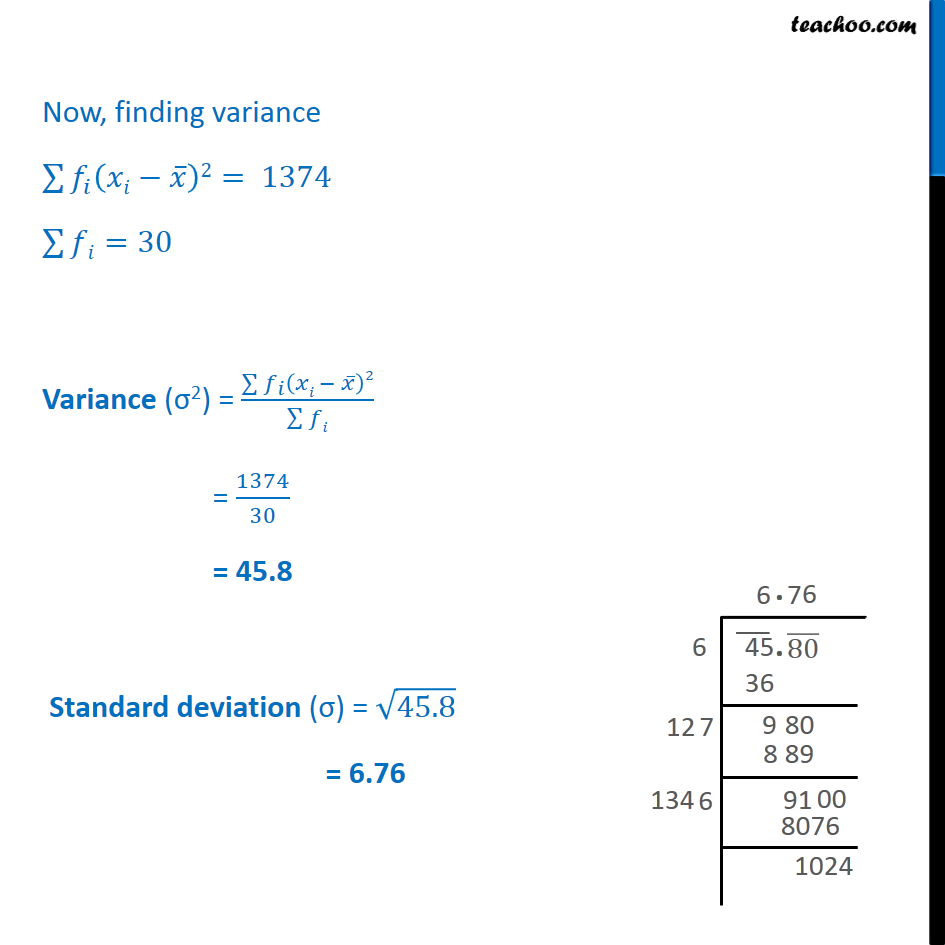Standard deviation and variance - Discrete frequency (grouped data)

Chapter 13 Class 11 Statistics
Concept wiseLearn in your speed, with individual attention - Teachoo Maths 1-on-1 Class

### Transcript

Example 9 Find the variance and standard deviation for the following data: First we will calculate mean Now, finding variance 2 = 1374 =30 Variance ( 2) = 2 = 1374 30 = 45.8 Standard deviation ( ) = 45.8 = 6.76